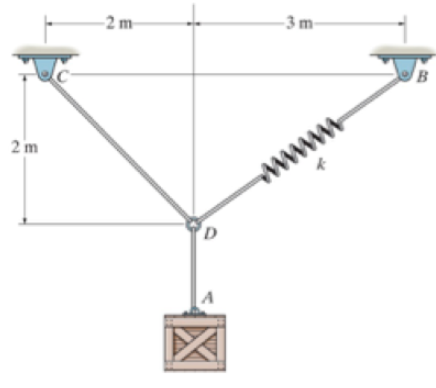# Problem: Determine the un-stretched length of DB to hold the 40kg crate in the position shown. Take k = 180N/m

###### FREE Expert Solution

Calculate the angles as follows:

${\mathbit{\theta }}_{\mathbf{1}}\mathbf{=}\mathbit{t}\mathbit{a}{\mathbit{n}}^{\mathbf{-}\mathbf{1}}\mathbf{\left(}\frac{\mathbf{2}}{\mathbf{2}}\mathbf{\right)}$

θ1 = 45°

${\mathbit{\theta }}_{\mathbf{2}}\mathbf{=}\mathbit{t}\mathbit{a}{\mathbit{n}}^{\mathbf{-}\mathbf{1}}\mathbf{\left(}\frac{\mathbf{2}}{\mathbf{3}}\mathbf{\right)}$

θ2 = 33.7°

Calculate the net force along the horizontal direction as:

$\begin{array}{rcl}\mathbf{\Sigma }{\mathbf{F}}_{\mathbf{x}}& \mathbf{=}& {\mathbf{T}}_{\mathbf{B}\mathbf{D}}\mathbf{c}\mathbf{o}\mathbf{s}{\mathbf{\theta }}_{\mathbf{2}}\mathbf{-}{\mathbf{T}}_{\mathbf{C}\mathbf{D}}\mathbf{c}\mathbf{o}\mathbf{s}{\mathbf{\theta }}_{\mathbf{1}}\mathbf{-}\mathbf{m}\mathbf{g}\\ \mathbf{0}& \mathbf{=}& {\mathbf{T}}_{\mathbf{B}\mathbf{D}}\mathbf{c}\mathbf{o}\mathbf{s}\mathbf{\left(}\mathbf{33}\mathbf{.}\mathbf{7}\mathbf{\right)}\mathbf{-}{\mathbf{T}}_{\mathbf{C}\mathbf{D}}\mathbf{c}\mathbf{o}\mathbf{s}\mathbf{\left(}\mathbf{45}\mathbf{\right)}\mathbf{-}\mathbf{\left(}\mathbf{40}\mathbf{\right)}\mathbf{\left(}\mathbf{9}\mathbf{.}\mathbf{8}\mathbf{\right)}\\ {\mathbf{T}}_{\mathbf{B}\mathbf{D}}\mathbf{c}\mathbf{o}\mathbf{s}\mathbf{\left(}\mathbf{33}\mathbf{.}\mathbf{7}\mathbf{\right)}& \mathbf{=}& {\mathbf{T}}_{\mathbf{C}\mathbf{D}}\mathbf{c}\mathbf{o}\mathbf{s}\mathbf{\left(}\mathbf{45}\mathbf{\right)}\mathbf{+}\mathbf{392}\\ {\mathbf{T}}_{\mathbf{B}\mathbf{D}}& \mathbf{=}& \frac{\mathbf{392}}{\mathbf{s}\mathbf{i}\mathbf{n}\mathbf{\left(}\mathbf{33}\mathbf{.}\mathbf{7}\mathbf{\right)}\mathbf{+}\mathbf{c}\mathbf{o}\mathbf{s}\mathbf{\left(}\mathbf{45}\mathbf{\right)}\left[\frac{cos\left(33.7\right)}{cos\left(45\right)}\right]}\end{array}$

TBD = 282.7 N

79% (211 ratings)###### Problem Details

Determine the un-stretched length of DB to hold the 40kg crate in the position shown. Take k = 180N/m